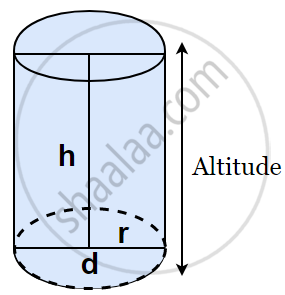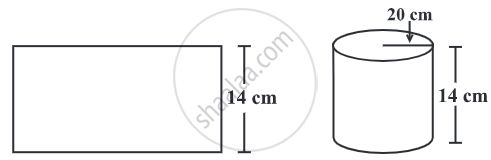# Volume of a Cylinder

## Formula

• Volume of a Cylinder = πr2h.

## Notes

### Volume of aCylinder:The volume of a cylinder can be obtained as: base area × height

= area of circular base × height = πr2h

So, the Volume of a Cylinder = πr2h, where r is the base radius and h is the height of the cylinder.

## Example

A rectangular paper of width 14 cm is rolled along its width and a cylinder of radius 20 cm is formed. Find the volume of the cylinder. (Take 22/7 for π)A cylinder is formed by rolling a rectangle about its width. Hence the width
of the paper becomes height and the radius of the cylinder is 20 cm.
Height of the cylinder = h = 14 cm
Radius = r = 20 cm
Volume of the cylinder = V = πr2h
= 22/7 × 20 × 20 × 14
= 17600 cm3
Hence, the volume of the cylinder is 17600 cm3.

## Example

A rectangular piece of paper 11 cm × 4 cm is folded without overlapping to make a cylinder of height 4 cm. Find the volume of the cylinder.
Length of the paper becomes the perimeter of the base of the cylinder and
width becomes height.
Let radius of the cylinder = r and height = h
Perimeter of the base of the cylinder = 2πr = 11
or 2 xx 22/7 xx r = 11
Therefore,
r = 7/4 cm
Volume of the cylinder = V = πr2h
= 22/7 xx 7/4 xx 7/4 xx 4 cm3
= 38.5 cm3
Hence the volume of the cylinder is 38.5 cm3.
If you would like to contribute notes or other learning material, please submit them using the button below.

### Shaalaa.com

Volume of the Cylinder [00:06:06]
S
0%